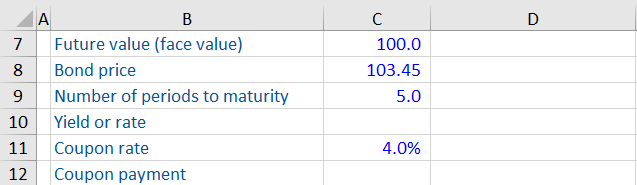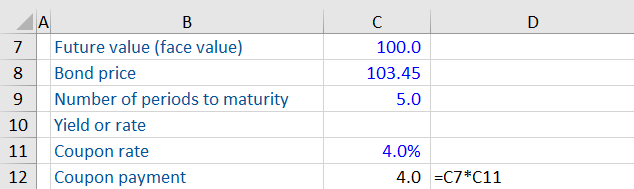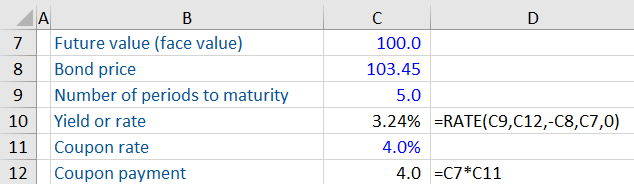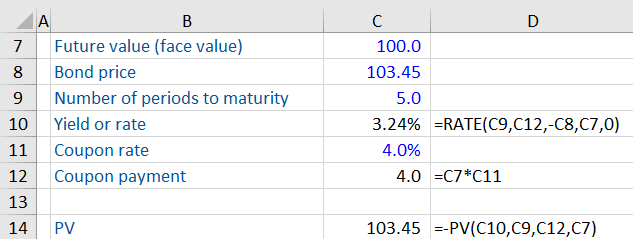## What are Bond Yields?

A bond’s yield is the return an investor realizes on a bond in percentage terms. It is effectively the interest rate a bond holder expects to receive on the bond. In practice, investors know the market price of a bond, and they want to know the yield to be realized at that price.

If a bond pays more than one cash flow (coupon), then there is no direct formula that can be used to calculate the bond’s yield. Instead, an iterative approach is used. This is referred to as the yield to maturity (YTM).

## Key Learning Points

• Bond yields refer to the annual return investors would realize by investing in a bond
• Yield to maturity is one of the most used yield types in the fixed income market
• There is no single formula for calculating bond yields. Instead, an estimate is calculated using an iterative process e.g. ”RATE” function in Excel
• The yield to worst is the lowest potential return an investor can get from investing in a bond, assuming there is no default
• Bond prices and yields have an inverse relationship. When the price of a bond increases, the yield falls, and vice versa
• Nominal yields do not factor in inflation. Real yields are bond yields adjusted for inflation

## How to Calculate Bond Yield

The price of a bond is the sum of its present value of future cash flows (outstanding coupon payments and the redemption payment). The present value is calculated using a discount rate, which equals the yield. We have covered the steps involved in calculating bond prices in detail in our blog on bonds.

However, if you know the price, there is no single formula for calculating the discount rate or the (bond yield). The yield is calculated using an iterative process. The discount rate is sensitized until the sum of the present value of the future cash flows matches the current bond price. The discount rate, in this case, is known as the yield to maturity (YTM).

We can calculate bond yields using the RATE function in Excel. This feature takes care of the iterations and calculates the bond yield in one go.

## Example: Bond Yield

An investor purchased a 5-year 4% coupon bond with annual payments at a price of 103.45%. What is the yield to maturity of this bond?The first step is to calculate the coupon payment of the bond. The coupon is the rate of interest paid to the bondholder. This is calculated as the face value multiplied by the coupon rate.The YTM is calculated with the RATE function in Excel. The function is summarized below:

RATE =(nper, pmt, [PV], type)

Where:

• Nper is the number of periods to maturity
• Pmt is the coupon payments per period
• PV is the present value i.e. the price of the bond (must be entered as a negative value)
• Type is the timing of the payment in the period denoted by either 0 (end) or 1 (beginning)

Using the RATE function gives the YTM for this bond at 3.24%:The PV function can be used as a sense check for the calculation because PV represents the price of the bond.

As can be seen, the present value of this bond is equal to 103.45 based on a coupon payment of 4.0, 5 years to maturity and an annual rate of return of 3.24%.The rate of a bond can also be calculated using the Goal Seek tool in Excel. This uses an iterative method to calculate the YTM based on a target number and set of assumptions.

## Bond Yields – Key Concepts

### Yield to Worst vs. Yield to Maturity

Some bonds have a clause where the issuer can call, or redeem, the bonds earlier than the maturity date, these would be known as Callable bonds. Take an example of a 4-year 3% coupon bond priced at 105. The bond has a call where the issuer can redeem the bond earlier (in year 2) by paying 101% of the face value. How will an investor calculate the yield on this bond?

In this case, the bond has two possible yields: Yield to maturity and yield to worst. If the bond is held to maturity (4 years), it will give a yield to maturity of 1.7%. If the bond is called earlier, it will give a yield of 0.99% (known as the yield to worst). The yield to worst is the lowest potential return an investor can get from investing in a bond, assuming there is no default.

### Nominal vs. Real Yield

Nominal yields indicate how much the amount invested in a bond will grow over time. However, nominal yields do not factor in inflation. If the nominal yield of a bond is 4% and inflation is 2%, the investor will get a yield of only 2%. This is known as the real yield or the bond’s yield adjusted for inflation.